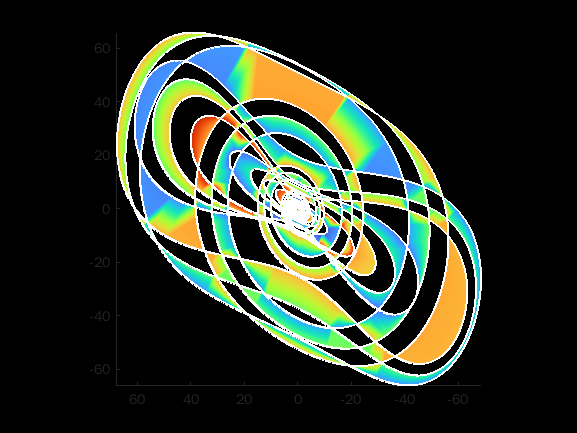• /

on 11 Oct 2021
• 9
• 89
• 2
• 0
• 265
t = 0:5e-5:10;
%% solving chaotic dynamics
[~,y]=ode15s(@f,t,[.1 0 0]);
%% plotting magic ;)
patch(y(:,1),y(:,2),-y(:,3),t,'EdgeC','w','linew',2);
set([gcf,gca],'Color','k');
colormap(flipud(turbo));
axis tight equal;
view(180,-90);%% ordinary differential equation
function w = f(t,X)
x = X(1);
y = X(2);
z = X(3);
w = [40*(y-x)+x*z/2; 20*y-x*z;.833*z+x*y-.65*x*x];
end
%% References:
%  Istvan. Strange Attractors visualisation of chaotic equation.
% url = https://chaoticatmospheres.com/mathrules-strange-attractors
% (Accessed on October 11, 2021).